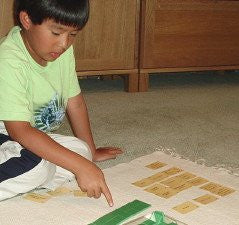• 39000

• Second grade materials for reinforcement
• Assorted formats. Most include answer sheets; cut materials include organization labels.
• Numerous exercise sets
Thousand Line & Labels, Order (blue), Order (pink), Order (green), Place Value (blue), Place Value (pink), Place Value (green), Numeration (blue), More Numeration (pink), Tens (gold), Exchange, Money B, Time B, Problem Solving B, Mixed Practice B, Thousands (green), Problem Solving C, Mixed Practice C, Geometry Introduction, Money C, Time C, Fraction Match, Roman Numerals, Problem Solving D, Mixed Practice D, Sets (Introduction), Sets (Union & Intersection), Fraction Concepts, Decimal Introduction